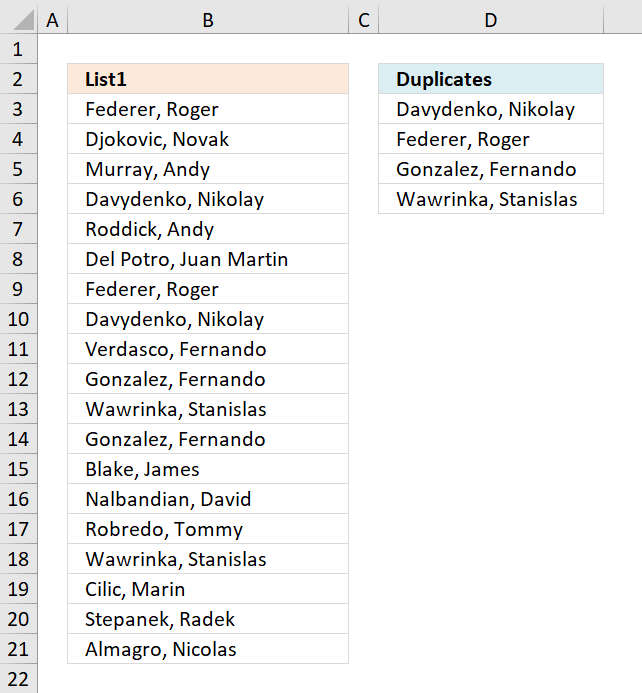Author: Oscar Cronquist Article last updated on November 22, 2018The following array formula extracts duplicate values sorted from A to Z from cell range B3:B21.

Excel array formula in C2:

=INDEX(\$A\$2:\$A\$20, MATCH(MIN(IF(COUNTIF(\$A\$2:\$A\$20, \$A\$2:\$A\$20)*IF(COUNTIF(C1:\$C\$1, \$A\$2:\$A\$20)=1, 0, 1)>1, COUNTIF(\$A\$2:\$A\$20, "<"&\$A\$2:\$A\$20), "")), IF(COUNTIF(\$A\$2:\$A\$20, \$A\$2:\$A\$20)>1, COUNTIF(\$A\$2:\$A\$20, "<"&\$A\$2:\$A\$20), ""), 0))

#### How to enter an array formula

1. Copy above formula
2. Double click on cell D3
3. Paste formula to cell D3
4. Press and hold CTRL + SHIFT simultaneously
5. Press Enter once
6. Release all keys

The formula now looks like this: {=arrayformula}

Don't enter the curly brackets yourself, they appear automatically.

### Explaining formula in cell C2

#### Step 1 - Count values

The COUNTIF fucntion counts values based on a condition or criteria, this way we can identify which values are duplicates and which are not.

COUNTIF(\$B\$3:\$B\$21,\$B\$3:\$B\$21)

becomes

COUNTIF({"Federer, Roger "; "Djokovic, Novak "; "Murray, Andy "; ... ; "Almagro, Nicolas "},{"Federer, Roger "; "Djokovic, Novak "; "Murray, Andy "; ... ; "Almagro, Nicolas "})

and returns

{2;1;1;2;1;1;2;2;1;2;2;2;1;1;1;2;1;1;1}.

#### Step 2 - Display first instance of each duplicate

The following COUNTIF function makes sure that the output list only contains unique values. The first argument contains a cell reference that expands as the formula is copied to cells below.

COUNTIF(D2:\$D\$2,\$B\$3:\$B\$21)<>1

becomes

COUNTIF("Duplicates",{"Federer, Roger "; "Djokovic, Novak "; "Murray, Andy "; ... ; "Almagro, Nicolas "})<>1

becomes

{0;0;0;0;0;0;0;0; 0;0;0;0;0;0; 0;0;0;0;0}<>1

and returns

{TRUE; TRUE; TRUE; TRUE; TRUE; TRUE; TRUE; TRUE; TRUE; TRUE; TRUE; TRUE; TRUE; TRUE; TRUE; TRUE; TRUE; TRUE; TRUE}.

#### Step 3 - Multiply arrays

Both values must return TRUE.

COUNTIF(\$B\$3:\$B\$21,\$B\$3:\$B\$21)*(COUNTIF(D2:\$D\$2,\$B\$3:\$B\$21)

becomes

{2;1;1;2;1;1;2;2;1;2;2;2;1;1;1;2;1;1;1}*{TRUE; TRUE; TRUE; TRUE; TRUE; TRUE; TRUE; TRUE; TRUE; TRUE; TRUE; TRUE; TRUE; TRUE; TRUE; TRUE; TRUE; TRUE; TRUE}

and returns

{2; 1; 1; 2; 1; 1; 2; 2; 1; 2; 2; 2; 1; 1; 1; 2; 1; 1; 1}.

 Boolean Boolean Multiply FALSE FALSE 0 FALSE TRUE 0 TRUE TRUE 1

#### Step 4 - Replace TRUE with a number representing the order if list were sorted

The IF function returns a value based on a logical expression, if TRUE the second argument is returned, if FALSE the third argument.

IF(COUNTIF(\$B\$3:\$B\$21,\$B\$3:\$B\$21)*(COUNTIF(D2:\$D\$2,\$B\$3:\$B\$21)<>1)>1,COUNTIF(\$B\$3:\$B\$21,"<"&\$B\$3:\$B\$21),"")

becomes

IF({2; 1; 1; 2; 1; 1; 2; 2; 1; 2; 2; 2; 1; 1; 1; 2; 1; 1; 1}>1,COUNTIF(\$B\$3:\$B\$21,"<"&\$B\$3:\$B\$21),"")

becomes

IF({TRUE; FALSE; FALSE; TRUE; FALSE; FALSE; TRUE; TRUE; FALSE; TRUE; TRUE; TRUE; FALSE; FALSE; FALSE; TRUE; FALSE; FALSE; FALSE},COUNTIF(\$B\$3:\$B\$21,"<"&\$B\$3:\$B\$21),"")

becomes

IF({TRUE; FALSE; FALSE; TRUE; FALSE; FALSE; TRUE; TRUE; FALSE; TRUE; TRUE; TRUE; FALSE; FALSE; FALSE; TRUE; FALSE; FALSE; FALSE},{7;6;11;3;14;5;7;3;16;9;17;9;1;12;13;17;2;15;0},"")

and returns

{7;"";"";3;"";"";7;3;"";9;17;9;"";"";"";17;"";"";""}.

#### Step 5 - Get smallest value in array

The MIN function returns the samllest value in a cell range or array.

MIN(IF(COUNTIF(\$A\$2:\$A\$20, \$A\$2:\$A\$20)*IF(COUNTIF(C1:\$C\$1, \$A\$2:\$A\$20)=1, 0, 1)>1, COUNTIF(\$A\$2:\$A\$20, "<"&\$A\$2:\$A\$20), ""))

becomes

MIN({7;"";"";3;"";"";7;3;"";9;17;9;"";"";"";17;"";"";""})

and returns 3.

#### Step 6 - Replace TRUE in array with a number representing the order if list were sorted

IF(COUNTIF(\$B\$3:\$B\$21,\$B\$3:\$B\$21)>1,COUNTIF(\$B\$3:\$B\$21,"<"&\$B\$3:\$B\$21),"")

becomes

IF({TRUE; FALSE; FALSE; TRUE; FALSE; FALSE; TRUE; TRUE; FALSE; TRUE; TRUE; TRUE; FALSE; FALSE; FALSE; TRUE; FALSE; FALSE; FALSE}, COUNTIF(\$B\$3:\$B\$21,"<"&\$B\$3:\$B\$21),"")

becomes

IF({TRUE; FALSE; FALSE; TRUE; FALSE; FALSE; TRUE; TRUE; FALSE; TRUE; TRUE; TRUE; FALSE; FALSE; FALSE; TRUE; FALSE; FALSE; FALSE}, {7;6;11;3;14;5;7;3;16;9;17;9;1;12;13;17;2;15;0},"")

and returns {7;"";"";3;"";"";7;3;"";9;17;9;"";"";"";17;"";"";""}.

#### Step 7 - Return relative position

MATCH(MIN(IF(COUNTIF(\$B\$3:\$B\$21,\$B\$3:\$B\$21)*(COUNTIF(D2:\$D\$2,\$B\$3:\$B\$21)<>1)>1,COUNTIF(\$B\$3:\$B\$21,"<"&\$B\$3:\$B\$21),"")),IF(COUNTIF(\$B\$3:\$B\$21,\$B\$3:\$B\$21)>1,COUNTIF(\$B\$3:\$B\$21,"<"&\$B\$3:\$B\$21),""),0)

becomes

MATCH(3, {7;"";"";3;"";"";7;3;"";9;17;9;"";"";"";17;"";"";""},0)

and returns 4.

#### Step 8 - Return value

The INDEX function returns a value from a cell range based on a row and column number. The cell range is a single column so the column number is not neccessary.

INDEX(\$A\$2:\$A\$20, MATCH(MIN(IF(COUNTIF(\$A\$2:\$A\$20, \$A\$2:\$A\$20)*IF(COUNTIF(C1:\$C\$1, \$A\$2:\$A\$20)=1, 0, 1)>1, COUNTIF(\$A\$2:\$A\$20, "<"&\$A\$2:\$A\$20), "")), IF(COUNTIF(\$A\$2:\$A\$20, \$A\$2:\$A\$20)>1, COUNTIF(\$A\$2:\$A\$20, "<"&\$A\$2:\$A\$20), ""), 0))

becomes

INDEX(\$A\$2:\$A\$20, 4)

and returns "Davydenko, Nikolay " in cell D3.